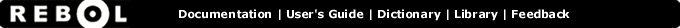# Max - Function Summary

## Summary:

Returns the greater of the two values.

## Usage:

max value1 value2

## Arguments:

value1 - The value1 argument. (must be: number pair char money date time tuple series)

value2 - The value2 argument. (must be: number pair char money date time tuple series)

## Description:

Returns the maximum of two values.

 ``` print max 0 100 100```

 ``` print max 0 -100 0```

 ``` print max 4.56 4.2 4.56```

The maximum value is computed by comparison, so MAX can also be used for non-numeric datatypes as well.

 ``` print max 1.2.3 1.2.8 1.2.8```

 ``` print max "abc" "abd" abd```

 ``` print max 12:00 11:00 12:00```

 ``` print max 1-Jan-1920 20-Feb-1952 20-Feb-1952```

Using MAX on XY pairs will return the maximum of each X and Y coordinate separately.

 ``` print max 100x10 200x20 200x20```

## Related:

< - Returns TRUE if the first value is less than the second value.
> - Returns TRUE if the first value is greater than the second value.
maximum - Returns the greater of the two values.
maximum-of - Finds the largest value in a series
min - Returns the lesser of the two values.